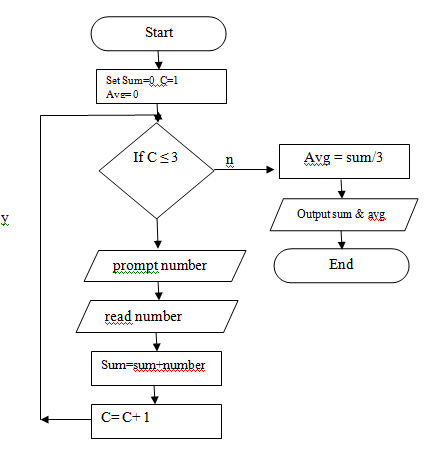# Example flowchart 1: Find the result of addition of three numbers. What about their average?Pseudo-code:

```1 Start
2 Set Sum=0  C=1  Avg= 0
3 If C ≤ 3
a Yes, go to step 4
b No go to step 8
4 Input number
5 Sum= sum+ number
6 C= C+ 1
7 Go to step 3
8 Avg = sum/3
9 Output sum, avg
10 End
```